#Function Repository Resource:

# ToNegabinary

Get the negabinary representation of an integer

Contributed by: Eric W. Weisstein
 ResourceFunction["ToNegabinary"][n] gives the negabinary representation of the integer n.

## Details and Options

Negabinary is a representation of an integer in base -2.

## Examples

### Basic Examples

Get the representation for 17 in negabinary:

 In:=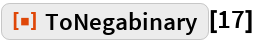Out=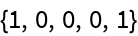From this representation, recover the original integer:

 In:=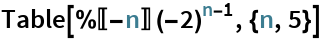Out=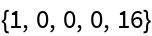In:=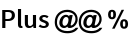Out=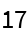Using FromNegabinary:

 In:=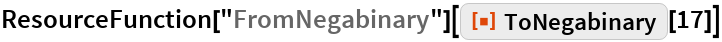Out=Some integers have the same representation in base 2:

 In:=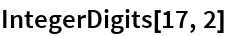Out=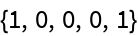Plot the negabinary representation for the first 22 integers:

 In:=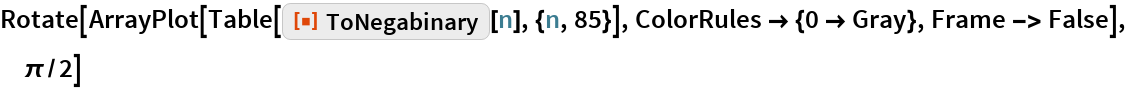Out=In:=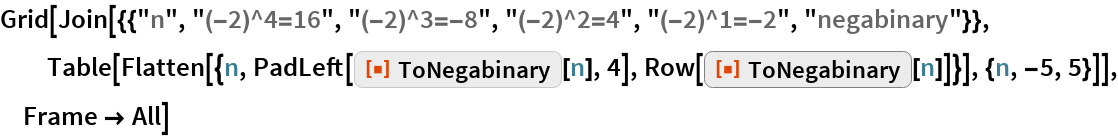Out=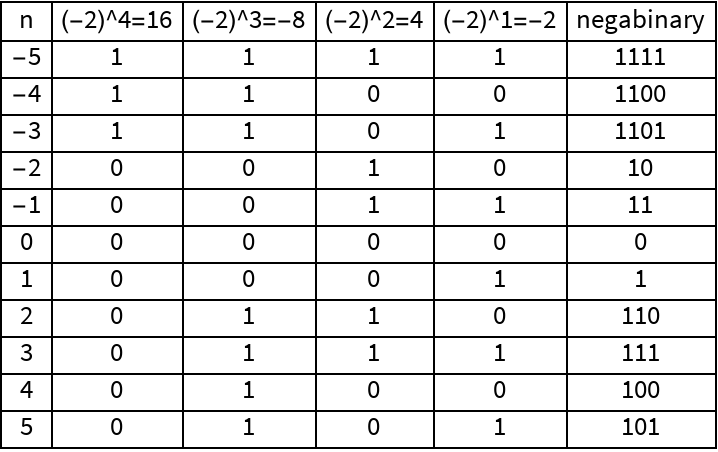### Scope

ToNegabinary handles negative numbers:

 In:=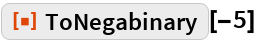Out=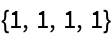## Requirements

Wolfram Language 11.3 (March 2018) or above